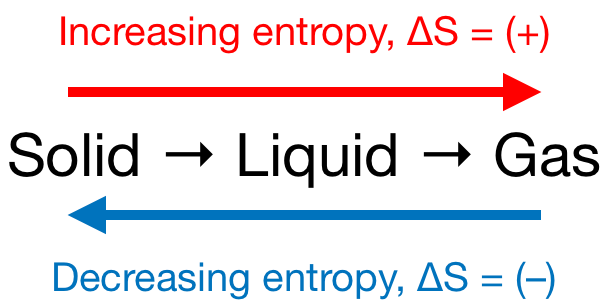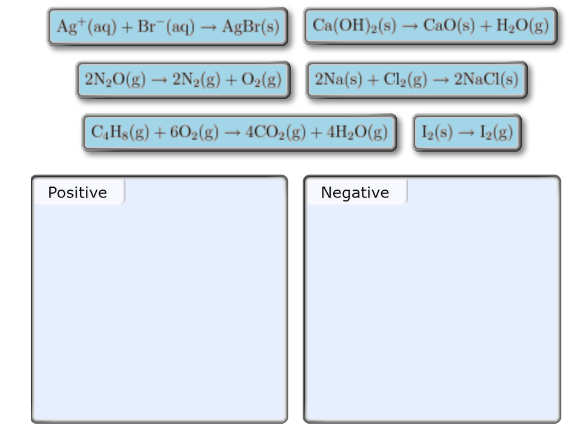# Problem: Predict the sign of the entropy change, ΔS°, for each of the reaction displayed. Drag the appropriate items to their respective bins.

🤓 Based on our data, we think this question is relevant for Professor Randles' class at UCF.

###### FREE Expert Solution

We’re being asked to predict the sign of the entropy change (ΔS) for the given reactions. Recall that if:

• ΔS > 0 or ΔS = (+); entropy is increased

• ΔS < 0 or ΔS = (–); entropy is decreased

Remember that phase changes lead to a change in entropy:Also, recall that an increase in the number of gas molecules in the reaction also leads to an increase in entropy.###### Problem Details

Predict the sign of the entropy change, ΔS°, for each of the reaction displayed. Drag the appropriate items to their respective bins.What scientific concept do you need to know in order to solve this problem?

Our tutors have indicated that to solve this problem you will need to apply the Entropy concept. You can view video lessons to learn Entropy. Or if you need more Entropy practice, you can also practice Entropy practice problems.

What is the difficulty of this problem?

Our tutors rated the difficulty ofPredict the sign of the entropy change, ΔS°, for each of the...as medium difficulty.

How long does this problem take to solve?

Our expert Chemistry tutor, Dasha took 4 minutes and 20 seconds to solve this problem. You can follow their steps in the video explanation above.

What professor is this problem relevant for?

Based on our data, we think this problem is relevant for Professor Randles' class at UCF.